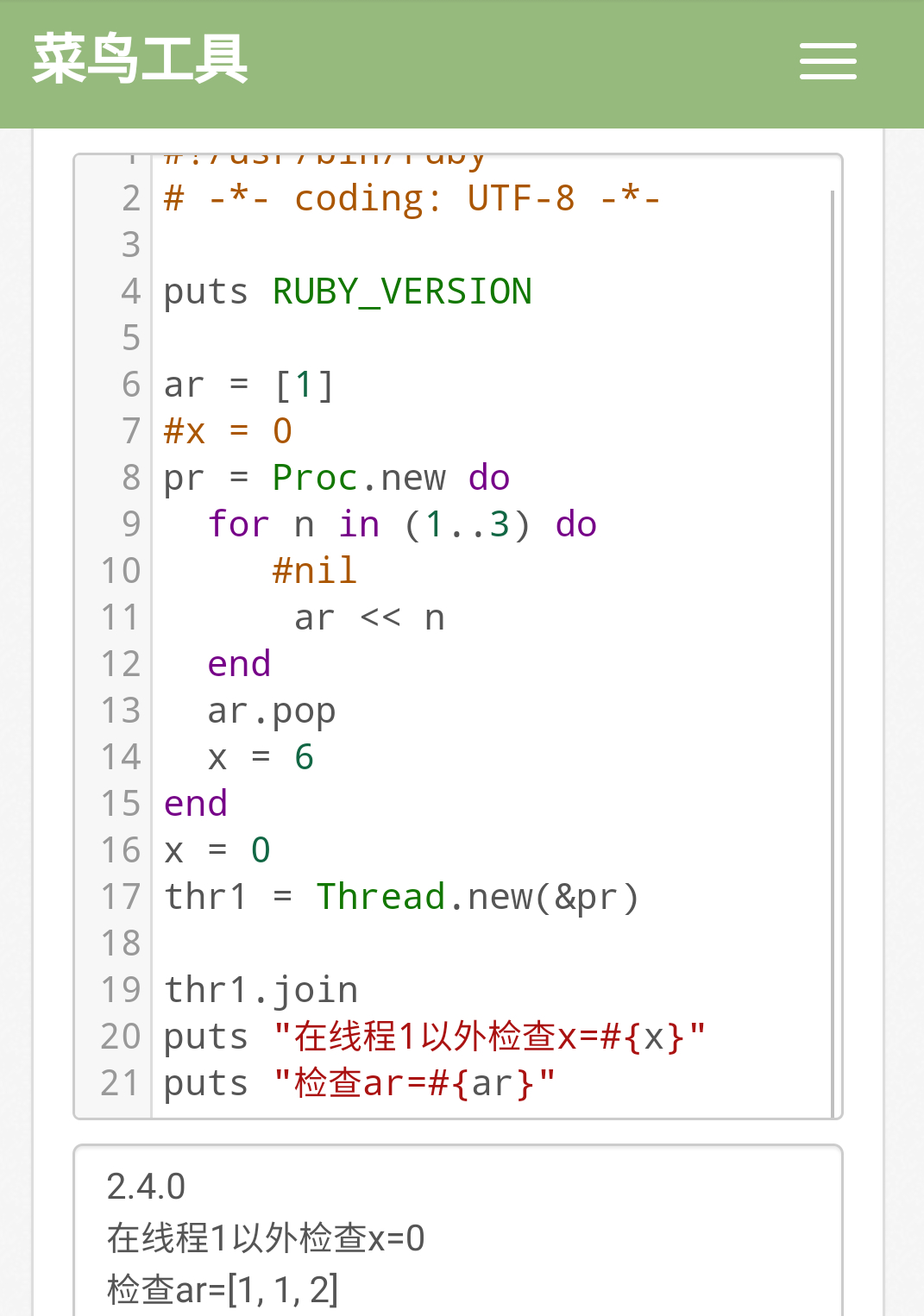# 新手问题 Proc 以内变量继承外部变量的问题

u4crella · 2020年03月27日 · 最后由 oatw 回复于 2020年03月31日 · 2811 次阅读``````x = 1
pr = Proc.new do
begin
x
rescue NameError
puts '未定义x'
end
begin
y
rescue NameError
puts '未定义y'
end
end
y = 2
puts 'proc定义完毕'
pr.call

# 结果是： 未定义y
``````

``````x = 1
pr = Proc.new do
(x+y)
end
y = 2
puts 'proc定义完毕'
puts pr.call
``````
``````x = 1
lam = lambda { return (x+y) }
y = 2
puts lam.call
``````
``````# python3 闭包1

x = 1
def f():
def g():
return (x+y)
return g()

y = 2
print(f())
``````
``````# python3 闭包2

x = 1
lam = lambda : (x+y)
y = 2
print(lam())
``````

You’re probably wondering where the block picks up its bindings. When you define the block, it simply grabs the bindings that are there at that moment, and then it carries those bindings along when you pass the block into a method.

``````class MyClass
def my_method
"original my_method()"
end

def another_method
my_method  # using has not been called, so still the original my_method
end
end

module MyClassRefinement
refine MyClass do
def my_method
"refined my_method()"
end
end
end

using MyClassRefinement
MyClass.new.my_method       # => "refined my_method()"
MyClass.new.another_method  # => "original my_method()"
``````